# Proof: A Brief Historical Survey

##### Age 14 to 18

Published 2008

A proof of a mathematical statement is a logical argument that shows the statement is true according to certain accepted standards.

The idea of proving a statement is true is said to have begun in about the 5th century BCE in Greece where philosophers developed a way of convincing each other of the truth of particular mathematical statements. They had to agree definitions of certain basic ideas (e.g. point, line, surface), and axioms [see note 1 below ] which were statements about the starting points (e.g. that it is possible to draw a circle of any radius). Over time these ideas and many others were developed in geometrical form, and finally collected and organised in thirteen Books by Euclid (325-263 BCE) in what was called his "Elements of Mathematics".

Ideally, the proof of a statement in any particular branch of mathematics uses the rules, definitions, axioms and theorems of that branch of mathematics, together with the rules of logic. Even though arithmetic, algebra and geometry each have different rules and procedures, we use the same kind of logic for each of them.

### Direct and Indirect Proof.

Direct Proof
Direct Proof is possible if we have agreed axioms and definitions to start from and an agreed method (a logical argument) that enables us to proceed logically step by step from what we know to what we do not know, but think is true.For example, propositions 5 and 6 in Euclid Book I are about the equality of the base angles of an isosceles triangle [see note 2 below ]. This is "obviously" true, but still has to be proved from first principles.

For some more difficult problems mathematicians developed a method of "working backwards". This works by assuming that the desired result is true, and showing that the consequences of that assumption are consistent with known facts and the basic principles. The final proof still had to be written out in the correct order.

Constructive proof is another form of direct proof. This is where an object has to be directly constructed from the basic elements of the system. There are many constructive proofs in Euclid Book I. Proposition 1 for example, shows how to construct an equilateral triangle [see note 3 below ].

However, it is not always possible to prove something by keeping to the strict rules of direct proof, and so mathematicians devised forms of indirect proof to achieve results.

Indirect Proof
Indirect proof means that we try to find a way of obtaining a result in some "round about" way. One way is by supposing that if the result we are looking for is not true, then the starting point cannot be true. [see note 4 below ]

Reductio ad Absurdum or Reduction to the Absurd [see note 5 below ] is another method of indirect proof where we try to show the opposite of the proposition to be true, but as soon as we come to a situation in the argument that we know is impossible, then we can say that the original proposition was true.

The proof that the square root of two is not rational [see note 6 below ] is a classic problem where an Indirect Proof is used. A proof originally attributed to Aristotle (384-322 BCE), uses basic ideas of arithmetic. This proposes that the diagonal of a square can be represented by a rational fraction, and produces an argument that constructs a number that is both even and odd (which is not possible of course).

In the 5th and 6th centuries BCE, the Pythagoreans [see note 7 below ] were interested in finding a common measure for the side of a square and its diagonal and discovered that these two lengths were incommensurable [see note 8 below ] by using a geometrical construction. In geometrical terms, this can be demonstrated by the successive subtraction of the smaller length from the larger:
In the red square in Figure I, BD = AB (=AC) and if BC (the diagonal) and AC (the side) have a common measure it must also be a common measure for CD = BC-BD.
Now AB' = B'D = DC
And B'C = AC - AB'. So B'C must also have the same common measure.
So, in the green square, the diagonal B'C and the side DC have the same common measure. By the same argument as for the red square, DC' minus DD' leaves D'C' (which again has the same common measure). This means that no matter what the size of the square, the common measure will always be the same. [See note 9 below ]
This is an indirect proof, because it shows that the process goes on forever producing a lower limit which is zero - contradicting the idea that a common measure can be found.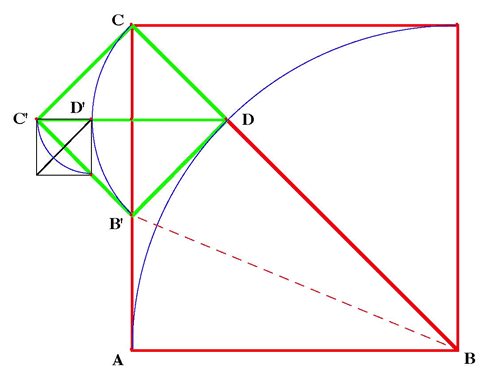Diagram I
In order to overcome some of the difficulties experienced by the Pythagoreans, Euclid ( in Book X of his Elements) showed how to manage the mathematics of incommensurables like $\sqrt 2, (3 + \sqrt 5)$ , etc, [See note 10 below ] purely by geometrical constructions.

### Keeping to the rules?

However, even in Euclid's Book I, the first proposition about congruent triangles [See note 11 below ], there were unsubstantiated assumptions. Euclid used the idea of "superposing" one triangle on another by a kind of transformation, but there are no postulates or definitions about what is meant by this action. In other words, even Euclid used an idea that was not fully justified.

It is now recognised that Archimedes (287-212 BCE) developed his own methods for calculating difficult results about areas and volumes enclosed by curves and surfaces, and then rewrote them in terms of the standard geometrical procedures of the time [See note 12 below ]. In a letter to a friend he said,
"I set myself the task of communicating to you a certain geometrical theorem which had not been investigated before but has now been investigated by me, and which I first discovered by means of mechanics and then exhibited by means of geometry."
In his first proof of the area of a parabolic segment, Archimedes used his discovery of the properties of the lever to balance the weight of a segment of a parabola against the weight of a triangle. In his second proof he used the method of exhaustion to show that the area of the segment was $\frac{4}{3}$ that of the triangle.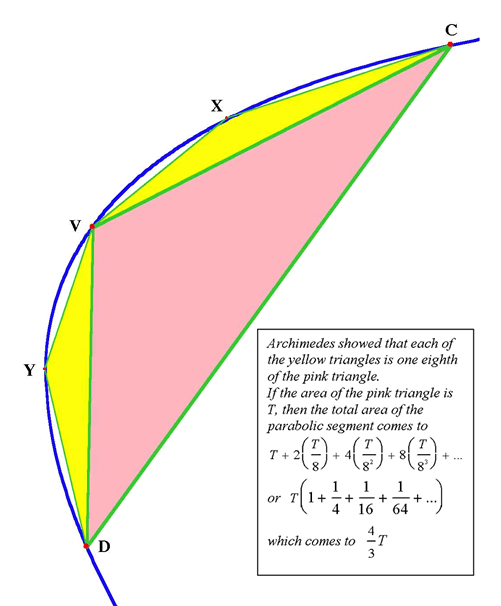So, the ideal proof methods set up by the earliest European mathematicians were not always adhered to, and later we will see that other mathematicians were still breaking the rules in order to achieve new results.

In the early ideas of the calculus, Cavalieri (1598-1647) and others adapted Archimedes' method of exhaustion to develop the method of "indivisibles". These were conceived of as infinitely small elements of lines, surfaces or volumes. Arguing by analogy, a line was made up of points, a surface was made from lines, as in the weave of cloth, and a volume thought of as a pile of very thin sheets of paper. In this way, areas and volumes of new objects could be compared with areas and volumes of known objects by a process of transformation.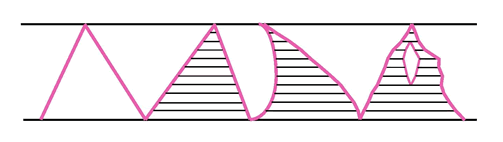For example, the area of a triangle was thought of as a very large number of very thin parallel lines. These were called 'Line Infinitesimals'. Areas of triangles on the same base and between the same parallels are equal. If each line remains the same total length, then the areas of the irregular shapes are the same as the area of the triangle s.

Throughout the 17th century, "proof by demonstration" was common.
The general idea was, "If I show you enough examples of how the algorithm works, then you will be convinced'.
Mathematicians demonstrated how they achieved their results in the early calculus by showing diagrams of curves, and sufficient examples of the procedures to convince their audience. In most cases the truth of the result was established by it's practical application, or that it achieved the same results as already known by a geometrical method. These experiments and demonstrations aided the discovery of interesting and important results, but did not conform to the strict standards of proof because they were based on unjustified assumptions about the very small quantities eventually "disappearing".

Many mathematicians were uncomfortable with this situation, but a logical basis for the calculus methods did not appear until the middle of the 19th century.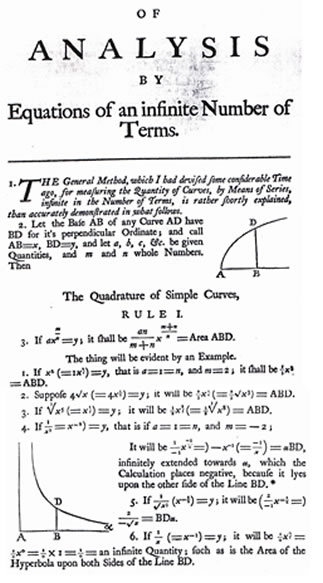This is part of the first English Edition of Newton's method for discovering the areas under curves using his method of infinite series published in 1745. This is the general rule for what we now call 'integration' in the calculus. Newton gives examples to demonstrate how it works with different algebraic expressions. .

When mathematicians like La Hire (1640-1718) and Chasles (1793-1880) began to develop projective geometry, they used a method called "projection and section" to show that many Euclidean proofs were also valid in the new geometry. For example, it was well known that the ellipse could be obtained by taking a section through a cone, so why not think of the ellipse as a transformation of a circle and look for the properties which remain the same? [See note 13 below ]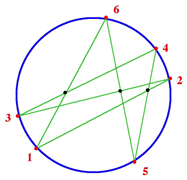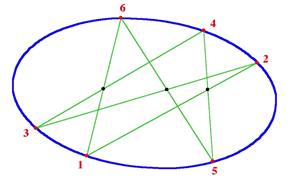For example, making an oblique cut on a circular cone produces an ellipse. By the method of 'Projection and Section' we can imagine the ellipse as the 'shadow' of the circle on a plane. Brianchon's (1783-1864) theorem states that if six vertices of a hexagon on a circle are joined as in the diagram, the intersections of the chords joining the points as shown lie in a straight line. The same result is also true for the ellipse or any other conic section. This is related to Pascal's theorem where the three points of intersection of the opposite sides of a hexagon lie in a straight line.

Imre Lakatos, in his Proofs and Refutations tells the story of the way the proof of the Descartes-Euler conjecture (F + V = E + 2) about counting the Faces, Vertices and Edges of polyhedra [see note 14 below ] was challenged by scientists working in the new field of crystallography, and how the original definition of a polyhedron had to be changed [see note 15 below ]. For example, it is possible to grow isomorphic [see note 16 below] crystals, one 'inside' another and there is a good argument to suggest that the conjecture might not be valid in this case. There are many examples where counterexamples of mathematical propositions have come from the physical world and mathematicians have had to re-think their theories.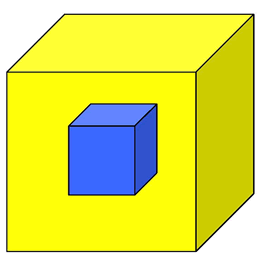The blue cube and the yellow cube are isomorphic. Can this be one polyhedron or two? So F + V = E + 4? What about a tetrahedron inside another tetrahedron?

In recent times, computers have been used to investigate solutions of difficult mathematical problems and one was used to tackle the proof of the famous Four Colour Theorem using a program to enumerate all possible maps [see note 17 below ]. The big question was since all proofs up to this time had been proved by people, was the fact that it had been completed by a computer a valid proof or not, and how could anyone be sure the computer had covered all the possible cases? This kind of counting procedure is called proof by enumeration or proof by exhaustion .

Proof by experiment or showing examples has always been a problem, as we have seen with the polyhedra and the maps, since we never know whether we have all the possibilities or when somebody will discover a counterexample. We need clear definitions and logical argument based on agreed facts to provide a sound proof.

Today, mathematicians have many ways of tackling proofs by using one system to help them find results in another. For example, Pythagoras' Theorem can be regarded as an arithmetic relation, an algebraic generalisation, a formula in trigonometry, or as geometric property. Each of these approaches gives different insights into the wide range and application of the general idea.
For this to work, it is important to be sure that the fundamental properties remain invariant as we transform the problem from one system to another.

### Wise Men from the East

Recently, important research in ancient Hindu and Chinese mathematics has uncovered some very different attitudes to "proving" results. Besides making their own original contributions, mathematicians wrote Commentaries on the works of earlier writers to elaborate ideas, to find other ways of achieving results, and of making new and useful applications. It is now clear that other cultures have produced ways of finding "truths" and generalisations quite different from Aristotle's logic.

Indian Mathematics
Many Indian writers who produced original mathematics, also took great pains to write commentaries on their own works and on works of earlier scholars. By the time of Bhaskaracharya (1114-1185) [see note 18 below ], Indian mathematicians had achieved an understanding of number systems and solution of equations which was well ahead of the Europeans. It is in their commentaries that we find detailed proofs and demonstrations of their results and discussion of appropriate methods. Until recently, much of this was obscured by superficial interpretation and misunderstandings of the cultural context [see note 19 below ].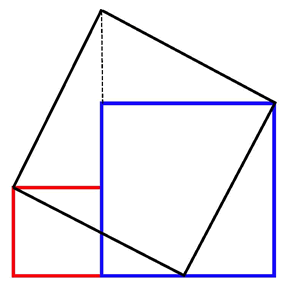An ancient Indian text, the 'Sulbasutra' (meaning 'Rope-Measuring') shows how to use two different size squares to find the area of a square which is the sum of the two areas. By discussing this diagram, students were able to justify and extend this method, thus discovering many geometrical properties much earlier than the Greek mathematicians .
Chinese Mathematics
The most important classic of Chinese mathematics is The Nine Chapters on the Mathematical Art which was written some time in the 1st century CE. Liu Hui (265-316 CE) wrote an important commentary on this work, and recent research shows us that there are other methods of investigating mathematical ideas other than the commonly accepted proof methods of Euclid. During this period in China, mathematics proceeded as a discussion between master and student who together focussed on the diagram; its appearances and possibilities. This shows that deduction from first principles is not the only model for the discovery and checking of mathematical results. This is a model of mathematical reasoning quite different from that of Euclid, but just as important and fundamental with the aim of generalisation rather than abstraction, and of deeper understanding rather than logical proof.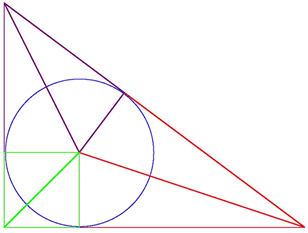This right-angled triangle comes from Lui Hui's commentary on the 'Nine Chapters'. By using some simple geometry students were able to find the area of the triangle and a number of other relations between the lines and the other areas in the diagram. This was done quite independently from the Greek geometers.

### Notes

1. The Greek word 'axiom' means an agreed starting point. The word 'postulate' is also used to describe 'what is possible', or what basic ideas can be used.
2. Proposition 5 used to be called the 'Pons Asinorum' (the Bridge of Asses) because it was once regarded as a test of understanding for the rest of the Elements.
3. This proof has been criticised because Euclid has no axiom which states that there is a point of intersection when two lines cross.
4. This is often called the contrapositive argument. i.e. If p implies q, then 'not q' implies 'not p'.
5. Some mathematicians choose to call 'reductio ad absurdum' orproof by contradiction, but others have decided it is a separate case.
6. A rational number is any one that can be represented by a fraction, like:
$$\frac{2}{3}\mbox{, }\frac{57}{21}\mbox{ or }\frac{3}{3}$$
7. Pythagoras (c.569-475 BCE) founded a society which continued well into the 5th century.
8. In-commensurable means that there is no common measure between two given lengths.In this case, a side of a square and its diagonal cannot be measured exactly in the same units, or fractions of the unit.
9. In this case, a side of a square and its diagonal cannot be measured exactly in the same units, or fractions of the unit. See Pedagogical Notes and Questions 1(c) .
10. Another name for these numbers is 'surds'.
11. For details on Euclid see D.E. Joyce's commentary at weblink EUC
12. See Netz and Noel (2007) for the most startling recent discoveries about Archimedes.
13. In this kind of projection, the lengths of lines and the shapes of curves change, but the order in which points are connected, remains the same.The term invariant refers to properties that remain the same under some kind of transformation.
14. The original conjecture by Descartes was investigated by Euler in 1758 when attempting to classify polyhedra.
15. The publication of Lakatos' original thesis in 1961 was a major factor in the development of Investigations in school mathematics.
16. Iso-morphic means the 'same shape'. Isomorphic crystals of chemicals with similar composition can grow on each another, as often seen in the school science laboratory.
17. See Robin Wilson's Four Colours Suffice (2002). This is the history of the map colouring problem first proposed by a student of Augustus De Morgan in 1852. The first proof in 1976 counted 1,936 different maps, but after re-testing the program and discovering mistakes, by 1994 the number had been reduced to 633. There are still some people who doubt the result.
19. In the early 20th century, Hardy, and others at Cambridge found many of the results of the brilliant Indian mathematician Ramanujan (1887-1920) difficult to understand because the proof methods were unlike anything they had seen before. Even today, mathematicians are still discovering important new results from the work of Ramanujan.

### References

Berggren, J. L. (2003) Episodes in the Mathematics of Mediaeval Islam New York, Berlin. Springer (original 1986)
D'Amore, B. (2005) Secondary school students' mathematical argumentation and Indian Logic (Nyaya).For the Learning of Mathematics 25 (2) July 2005 (26, 32)
Datta, B. ; Singh, A. N.(1935) History of Hindu mathematics, a source book. Lahore,: Motilal Banarsi Das. (Reprinted by Bharatiya Kala Prakashan, Delhi)
Dauben, J.W. (2007) Chinese Mathematics in Katz, V. J. (ed.) Sourcebook (187-384)
Lakatos, I. (1976) (Eds. Worrall, J. and Zahar, E.) Proofs and Refutations: The Logic of Mathematical Discovery London. Cambridge University Press
Kanigel, R. The Man Who Knew Infinity: A Life of the Genius Ramanujan Johns Hopkins Univerity Press
Katz, V. J. (2007) (ed.) The Mathematics of Egypt, Mesopotamia, China, India and Islam: A Sourcebook. Princeton and Oxford. Princeton University Press
Keller, Agathe (2005) Making diagrams speak, in Bhskara I 's commentary of the Aryabhatiya. Historia Mathematica 32: 275-302.
Keller, Olivier. (2006) Une Archeologie de la Geometrie (An Archeology of Geometry) Paris. Vuibert
Martzoff, J-C. (1987) A History of Chinese Mathematics Berlin, New York. Springer
Newton, Isaac. (1745) Sir Isaac Newton's Two Treatises on the Quadrature of Curves, and Analysis by Equations of an infinite Number of Terms explained: London John Stewart
Netz, R. and Noel, W (2007) The Archimedes Codex. London. Weidenfeld & Nicolson
Plofker, K. (2007) Mathematics in India in Katz, V. J., Sourcebook (385-514)
Li Yan and Du Shiran (1987) Chinese Mathematics : A Concise History. John N. Crossley, J.N. and Lun, A.W.C. Oxford. Oxford Science Publications.
Watson, A. and Mason, J. (1998) Questions and Prompts for Mathematical Thinking Derby. Association of Teachers of Mathematics
Wilson, R. (2002) Four Colours Suffice: How the Map Problem was Solved London. Penguin Books